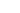### Tag: function pointer## More on function pointers

A function pointer is a pointer to a function, i.e. it’s a variable (a pointer) but instead of pointing to some data, it is assigned to a function. Most importantly, the compiler checks that the function you are assigning is compatible to that pointer. This is so, as I discussed earlier that when the function is executed, it correctly retrieves any parameters.

Here’s how you create a function pointer. Let’s start with a function that adds 1 to the input value.

``````int getValue(int x) {
return n + 1;
};
``````

This passes in x and returns an int. Let’s create a pointer to this function and this is the full program.

``````
#include <stdio.h>

int getValue(int n) {
return n + 1;
}

typedef int (*getValueFn)(int);

int main()
{
getValueFn f = &getValue;
printf( "Value of getValue = %d\n",f(1));
}
``````

It’s as simple as that. The complicated bit is turning the function signature into the typedef. Start with the function name. Put it in brackets and add a * at the start, and make the name different to the function you are assigning. It’s a new type. So getValue becomes (*getValueFn).

Now add on the return type at the start int and the parameters at the end (int n), but throw away the name n, we only need the type and you get int (*getValueFn)(int);

Finally add typedef, cook for 30 microseconds and you’re done! In the line getValueFn f = &getValue;
I’ve declared an instance of the type f and assigned to it the address of getValue. That gets called with a value f(1) and in this case returns 2.

It’s also good practice to check that the instance variable is not null before you call it.## Function pointers in C

The one thing worse for beginners than pointers is … function pointers. A normal pointer holds the address of another variable whereas a function pointer holds the address of some code.

But before I delve into the complexities of function pointers, you have to understand a little bit about functions. A function is defined, not just by what it does but the values you pass in and get back from it. Here are a couple of examples.

``````int add(int b,int c) {
return a + b;
}

void DoSomething(int value) {
//
}
``````

The code generated by the compiler has to pass in the parameters and usually does it by pushing values on the stack or in registers. In the code for the function, the first thing it does it fetch the parameters. So when you use a function pointer, according to the definition of that function pointer, the compiler will generate code in the function to extract parameters.

I’ll look at the syntax of function pointers tomorrow; it’s always the complicated bit, probably the most complicated bit but it dos give your program a bit more flexibility.

I’m now using the Prismatic plugin. Much nicer listing with indentations!